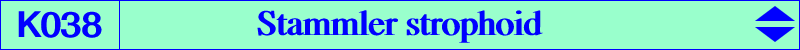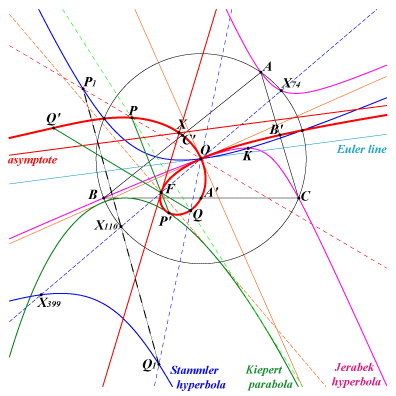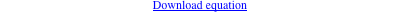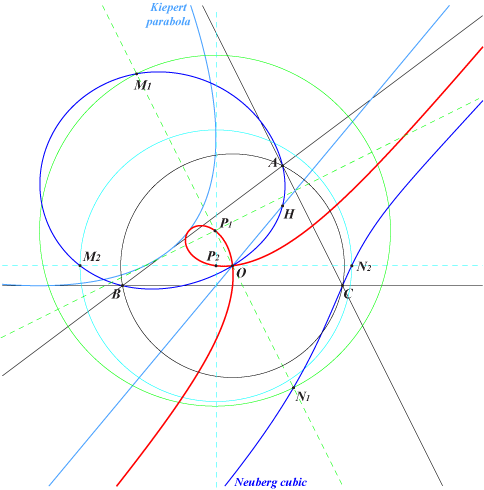too complicated to be written here. Click on the link to download a text file.X(3), X(30), X(36), X(131), X(187), X(1511), X(2482), X(3184), X(6150), X(6592), X(12095), X(12096), X(17729), X(35204), X(39162), X(39163), X(39164), X(39165), X(39987), X(47077), X(47078), X(47079), X(47080), X(47081), X(47082), X(47083), X(47084), X(47085), X(47086), X(47087), X(47088), X(47089) midpoints of ABC foci of the inscribed Steiner ellipse. inverses of the vertices of excentral and tangential triangles and more generally, inverses of all the points on the Stammler hyperbola.A'B'C' is the medial triangle. For any point P, denote by Pa, Pb, Pc its reflections in the sidelines BC, CA, AB. We know that PaPbPc and ABC are perspective if and only if P lies on the Neuberg cubic. On the other hand, PaPbPc and A'B'C' are perspective if and only if P lies on the Stammler strophoid. Furthermore, the perspector Q also lies on the curve and the line PQ is tangent to the Kiepert parabola. The node is O with tangents parallel to the asymptotes of the Jerabek hyperbola. The singular focus is F = X(1511) = midpoint of X(3)-X(110). The real asymptote is the homothetic of the Euler line under h(F, 2). It is the line X(30)X(125) which meets K038 again at X = X(39987), also on the line X(3)X(526). The Stammler strophoid is the inversive image of the Stammler hyperbola in the circumcircle : for P1 and Q1 antipodes on the Stammler hyperbola, their inversive images P and Q are conjugate points on the Stammler strophoid i.e. P and Q share the same tangential on the strophoid. K038 is the complement of K025, the Ehrmann strophoid. The homothetic of K038 under h(O, 2) is K725. See also Q071, the Darboux quintic. K038 is the Laplacian of the quintic Q047 i.e. the locus of points whose polar conic in the quintic is a rectangular hyperbola.A variable line L passing through O meets the Neuberg cubic again at two points M, N with midpoint P. The locus of P is the Stammler strophoid. The perpendicular at P to L is tangent to the Kiepert parabola. In other words, the Stammler strophoid is the pedal curve of O with respect to the Kiepert parabola. Compare with the Kiepert-Neuberg strophoid K591 where O is replaced by H. These two curves are two examples of Kiepert strophoids. More informations at K592 and K593. *** See also K009, locus property 2.